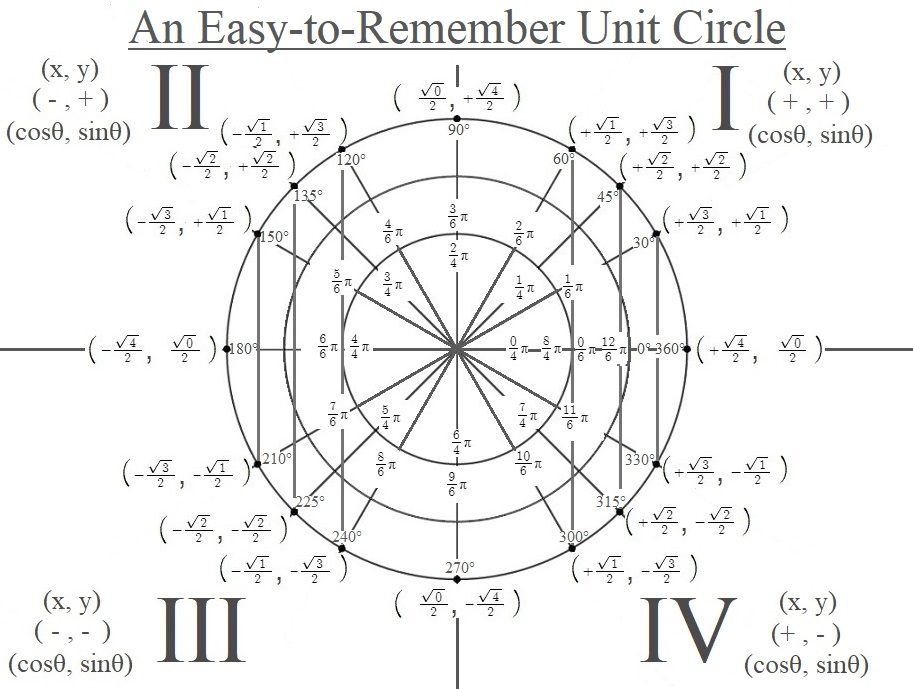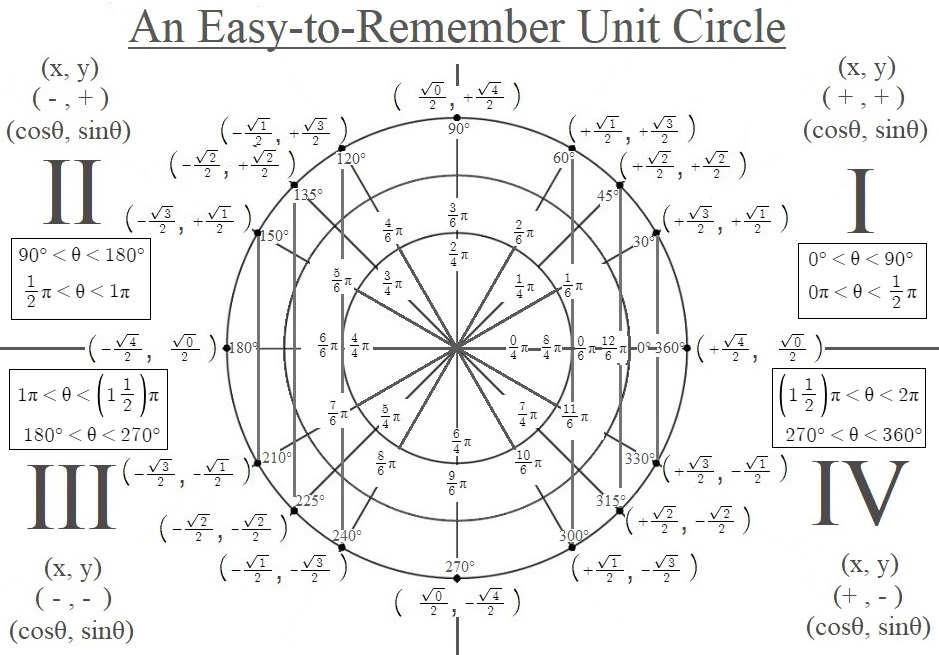# 1466 A Non-Simplified Yet Easy-to-Remember Unit Circle

Contents

### Look at the Patterns in this Unit Circle:

A unit circle has a radius of one and is often used to display the sines and cosines of special angles. I’ve made a unit circle that showcases the patterns that make memorizing how to complete one easier.

This unit circle is easy to memorize because many of the values have not been simplified. However, always remember to simplify the values when giving your final answer on any assignment or test!The patterns you see in the ordered pairs representing the cosines and sines are only coincidental: they are merely an aid somebody else created to make memorizing the values easier. Over half of the square roots can be simplified and should be. However, I do love them in their non-simplified form because it is easy to remember them, and it is easy to see the sine wave and the cosine wave as the square root values increase and decrease incrementally as we go around the unit circle.

I’ve also made a similar unit circle that might help students locate the angles in the quadrants better:### Factors of 1466:

• 1466 is a composite number.
• Prime factorization: 1466 = 2 × 733
• 1466 has no exponents greater than 1 in its prime factorization, so √1466 cannot be simplified.
• The exponents in the prime factorization are 1, and 1. Adding one to each exponent and multiplying we get (1 + 1)(1 + 1) = 2 × 2 = 4. Therefore 1466 has exactly 4 factors.
• The factors of 1466 are outlined with their factor pair partners in the graphic below.### More Facts about the number 1466:

1466 is the sum of two squares:
29² + 25² = 1466

1466 is also the hypotenuse of a Pythagorean triple:
216-1450-1466 calculated from 29² – 25², 2(29)(25), 29² + 25²

## 2 thoughts on “1466 A Non-Simplified Yet Easy-to-Remember Unit Circle”

1.adynt

This is really helpful! I can see you have worked extremely hard on this.
Hope you have the best day ever!

•ivasallay

Thank you! Yes, I did spend MANY hours working on it, but for me, it was all a pleasure.

This site uses Akismet to reduce spam. Learn how your comment data is processed.